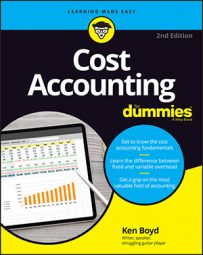##### Cost Accounting For DummiesIn cost accounting, revenue and production budgets forecast how many units you plan to produce and how many units you plan to sell.

Say you’re budgeting to manufacture garage doors. You need to forecast how many sales you expect. Then you consider how many garage doors you already have in inventory and plan how many you need to manufacture to meet the sales forecast. When you know the number of doors you need to make, you can budget for material and labor costs.

Direct costs are pretty straightforward, and you can use an activity level to assign indirect costs. Revenue, production, inventory, direct materials, direct labor, indirect costs (overhead), and cost of goods sold all are budgeted items.

## Apply the revenue formula in cost accounting

Say you forecast selling 200 garage doors in March. Consider how many garage doors you need to manufacture. Assuming a sales price of \$300 per door, here’s your revenue budget:

```Revenue budget = 200 units × \$300
Revenue budget = \$60,000```

## Use the inventory formula in cost accounting

```And now for the famous inventory formula:
Ending inventory = beginning inventory + production - sales```

Your production will change based on how many garage doors you already have in inventory. So if you already have 75 completed garage doors in beginning inventory, it’s clear that you won’t need to manufacture all of the 200 units you plan to sell.

But wait! Do you want any garage doors in ending inventory? If you think you’ll have orders during the first few days of the next month, you probably do. So maybe you decide on an ending inventory of 50 garage doors.

Take the inventory formula and calculate the garage door production you need. Assume x is production in units, and solve for x:

```Ending inventory = beginning inventory + production - sales
50 = 75 + x - 200
x = 175```

This simple algebra problem shows that production should be 175 units. This shows a production budget.

Garage Door Production Budget
Cost Quantity Price Total
Direct material (wood) 80 square feet \$1 per square foot \$80
Direct labor (labor) 2 hours \$25 per hour \$50
Indirect costs allocated 1 hour \$15 per hour \$15
Cost per unit \$145
Units Unit cost Total cost
Production cost 175 \$145 (above) \$25,375

The production budget includes direct materials, direct labor, and indirect costs (overhead). In this example, the indirect cost is allocated based on machine hours. Add the costs to get a unit cost. Then multiply units to be produced by the cost per unit. That amount is the total cost of production of \$25,375.

## Assess the cost of goods sold in cost accounting

The goods you produce for customers end up in one of two places: You either sell them (cost of goods sold), or they’re still on the shelf (finished goods inventory). Beginning inventory and production don’t matter.

What costs should be attached to the goods you sell? How much did they cost to produce? (If you’re a retailer, how much did they cost to get?) To continue with the garage door manufacturing example, assume that the first goods you sell are from beginning inventory. Because all 75 units of beginning inventory are sold, use a formula to determine how many units of the March production are sold:

```March production sold = total sales - beginning inventory
March production sold = 200 - 75
March production sold = 125```

Assume also that the cost per unit of beginning inventory is \$143. That cost is different from the March production cost of \$145. (Why the change? Because it seems like the costs of materials and labor to make a garage door go up all the time.) This table displays the cost of goods sold budget.

 Units Cost Per Unit Total Cost Beginning inventory 75 \$143 \$10,725 March production sold 125 \$145 \$18,125 Total 200 \$28,850

The total cost of goods sold is higher (\$28,850) than total production cost in the first table (\$25,375). That makes sense, because that table deals only with producing 175 units. You sold 200 units, but 75 units were from inventory. Because of adjustments for beginning and desired ending inventory, you don’t always need to produce in a month the number of units you sell in a month.

One more calculation. (There’s always one more calculation.) Now calculate your ending inventory budget:

```Ending inventory budget = units × per unit cost
Ending inventory budget = 50 × \$145
Ending inventory budget = \$7,250```

You’ve planned revenue, production, and inventory. Great! Now you need to figure out how to pay for it all. If you don’t have a budget for an adequate cash amount to operate, you can’t do business.